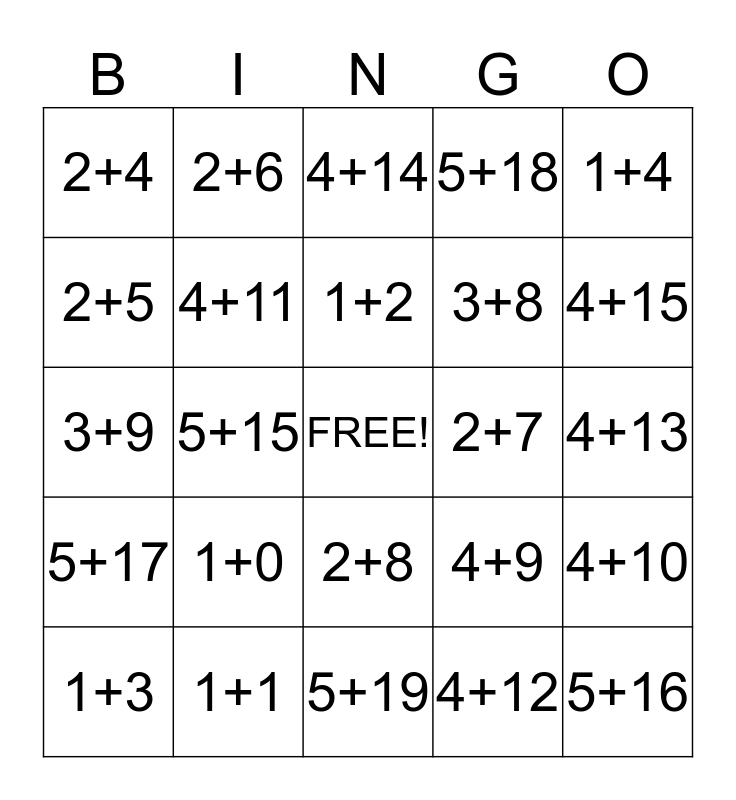This bingo card has a free space and 24 words: 1+0, 1+1, 1+2, 1+3, 1+4, 2+4, 2+5, 2+6, 2+7, 2+8, 3+8, 3+9, 4+9, 4+10, 4+11, 4+12, 4+13, 4+14, 4+15, 5+15, 5+16, 5+17, 5+18 and 5+19.

## Play Online